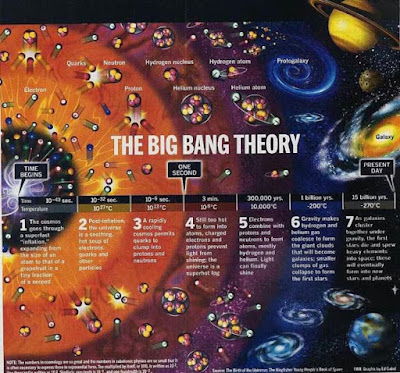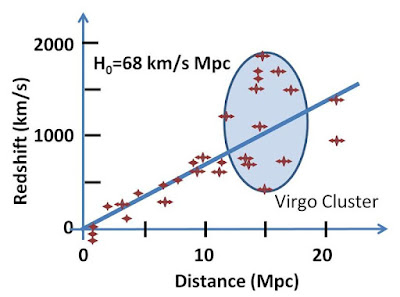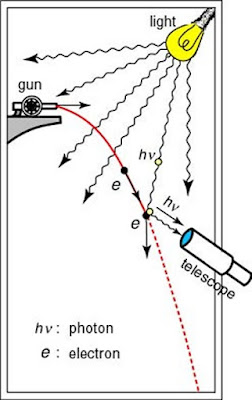# 8 Scientific Laws and Theories List you Really Should Know

Whatever we do, either we are launching a space exploration program else we are trying to discover or hypothesize or invent something on our planet earth; we need some fundamental laws and theories to synthesize a body of evidence or observations of a particular phenomenon. In this article, we’ll look at 8 scientific laws and theories list you really should know. Today we will start off with the Big Bang that how our universe works to the Heisenberg’s Uncertainty Principle that tells us that the concept of exact position and momentum together has no meaning in nature.

## 8. The Big Bang Theory#the big bang theory credit: imgur.com

Well in my view, everyone is quite aware of The Big Bang Theory. The Big Bang Theory is the current prevailing Cosmological Model For The Universe. Based on the research and combined work of Edwin Hubble, Georges Lemaitre, Albert Einstein, and so many others postulated that the universe began almost 13.8 billion years ago and is expanding outward till today. This 13.8 billion year is now well considered as the age of the universe. Once upon a time, the scientific community was divided between two cosmological models of the universe i.e the big bang theory and the steady-state theory. But after 1964, the big bang theory gained huge support from the scientific community when two American radio astronomers Arno Penzias and Robert Wilson accidentally discovered the Cosmic Microwave Background Radiation; a phenomenon that became the landmark evidence of the big bang origin of the universe.

## 7. Hubble’s Law Of Cosmic ExpansionHubble’s law of cosmic expansion credit: Wikimedia Commons

Its a fact that if Edwin Hubble would have not discovered Hubble’s law of cosmic expansion, then it is certain that we would have not the precise knowledge about the big bang theory of the universe. Hubble discovered that there are so many galaxies other than our home galaxy milky way. According to Hubble’s law of cosmic expansion, all the galaxies are moving away from our own and named the phenomenon as the recession.

The mathematical representation of Hubble’s Law Of Cosmic Expansion is stated as

V = H × d

where
V = Galaxy’s recessional velocity
= Hubble’s constant
d = Galaxy’s distance from the one which it’s being compared

✱ hubble’s constant is changing over time. the currently accepted value is 70 kilometers/second per megaparsec

## 6. Kepler’s Laws Of Planetary Motion

Till the 15th century, everyone believed in the Aristotelian-Ptolemaic Universe i.e the Geo-Centric Model Of The Universe. It states that the earth is stationary and at the center of the universe and each planet within our solar system revolves around the Earth. Finally, in the 16th-century scientific revolution overcome religious beliefs when Nicolaus Copernicus put forward his controversial Heliocentric Model Of The Universe stating that each planet including earth revolves around the Sun– not the Earth. But the Copernican revolution was not perfectly cemented in the history of the scientific world until the German mathematician, astronomer, and astrologer Johannes Kepler (with help of Tycho Brahe’s orbital calculation) enhanced the Nicolaus Copernicus heliocentric model of the universe by demonstrating that planets rather move in elliptical orbits, not in circular orbits as presented by Copernicus.Diagram of Kepler’s three laws with two planetary orbits Credit: Wikimedia Commons

Kepler’s Laws Of Planetary Motion for explaining the motion of planets around the Sun are as follow

1. The path of a planet is elliptical with the sun at one of the two foci.
2. A line segment joining a planet and the Sun sweeps out equal areas in equal intervals of time.
3. The square of the time period of a planet is proportional to the cube of the semi-major axis of its orbit.

## 5. Newton’s Law Of Universal Gravitation And Laws Of Motion

As long as we will talk about physics, as long as we will remember the name, sir Issac Newton. Almost 300 years ago, Issac Newton proposed a revolutionary idea; that any two objects, no matter what their size is, exert a gravitational force toward one another which is known to us as Newton’s law of universal gravitation. The mathematical representation is as followswhere
F = force between two masses
m1 = mass of the first object
m2 = mass of the second object
G = gravitational constant (6.674×10−11 N · (m/kg)2)
r = distance between the center of the two massesdiagram of two masses attracting one another Credit: Wikimedia Commons

Just because of Issac Newton’s law of universal gravitation scientists are able to calculate the gravitational pull between two objects and are able to put artificial satellites in orbit to detect weather etc.

let’s talk about newton’s other famous work i.e newton’s laws of motion. Issac Newton gave simple and easy-to-understand three laws of motion that are an essential component of Classical Physics.
The three laws of motion are as follow

1. In an inertial frame of reference, an object stays in motion unless acted upon by an outside force.
2. The acceleration of an object is given by the vector sum of all the forces divided by its mass (where mass remains constant)
3. For every action, there is an equal and opposite reaction.

## 4. Laws Of Thermodynamics

Well in the words of great Lord Kelvin— “Thermo-Dynamics is the subject of the relation of heat to forces acting between contiguous parts of bodies, and the relation of the heat to the electrical agency”. In fact, Scottish physicist Lord Kelvin was the first person to formulate a concise definition of thermodynamics. Thermodynamics is a branch of physics that deals with heat and temperature and their relation to energy and work. In simple terms, thermodynamics is the study of how energy works in a system, whether it’s a motor-bike engine or the earth’s core.Carnot heat engine working because of laws of thermodynamics Credit: Wikimedia Commons

It can be reduced to several basic laws; i.e four laws of thermodynamics, as follows
1.  Zeroth law of thermodynamics states that: if two systems are in thermal equilibrium with a third system, they are in thermal equilibrium with each other.
2. The first law of thermodynamics states that: the change in the internal energy of a system is equal to the difference between heat added to the system from its surroundings and work done by the system on its surroundings.
3. The second law of thermodynamics states that: in a natural thermodynamic process, the sum of the entropies of the interacting thermodynamic systems always increases
4. The third law of thermodynamics states that the entropy of a system approaches a constant value as the temperature of the system approaches absolute zero.

## 3. Archimedes buoyancy principle

One day, the greatest mathematician of classical antiquity Archimedes of Syracuse was about to take bath, when he himself entered a bathtub, he discovered that the water level increased when he entered the bathtub. After his discovery of what we today know as the Archimedes Principle, the Greek scholar was completely shocked that he didn’t even recognize that he was running naked on the streets of Syracuse yelling Eureka (a Greek word meaning “I found it”).Archimedes discovered the principle when he stepped into a bathtub and the water overflowed. Credit: Wikimedia Commons

Definition of Archimedes Buoyancy Principle in the words of Archimedes in his treatise On Floating Bodies and he quote

Any object, wholly or partially immersed in a stationary fluid, is buoyed up by a force equal to the weight of the fluid displaced by the object.

## 2. Einstein’s Theory Of General Relativity

This list would have not been complete if we had not included Albert Einstein’s theory of general relativity. Though there are so many works registered in the name of great Albert Einstein such as photoelectric effect, mass energy-equivalence, Brownian motion, and so on, Einstein’s theory of general relativity remains an essential and important discovery because it permanently altered how we look at the universe. Einstein showed equivalence between an inertial force of acceleration and force of gravity that space is curved (wrapped) and finite in size, predicting phenomenon that in today’s world known as length contraction, time dilation, gravitational lensing, black holes, etc.space-time lattice analogy Credit: Wikimedia Commons
In fact, Einstein’s concept of space-time curvature ( theory of general relativity) completely replaced Newton’s laws of universal gravitation from the heart of all the physicists around the globe. In the view of Issac Newton– there is an absolute frame of reference and within that absolute motion exists. But in the view of Albert Einstein– there is no absolute frame of reference. According to Einstien’s view or the general theory of relativity, everything within the universe depends on relative motion, and therefore, absolute motion ceases to exist.

## 1. Heisenberg’s uncertainty principle

When Albert Einstein gave his theory of relativity, physicists were baffled by the consequences of the theoretical science that how universal laws work like whether laws are rigid or able to bend and flex. Einstein’s theory made some astonishing discoveries and laid the foundation for Modern Physics as well as Quantum Physics but the confusion was introduced in theoretical physics. In the year 1927, this confusion led to the discovery of the uncertainty principle by German physicist Werner Heisenberg.

The uncertainty principle as in the words of Werner Heisenberg; and he quote

the concept of exact position and momentum together has no meaning in natureHeisenberg’s uncertainty principle Credit: Wikimedia Commons

In simple words, it is impossible to simultaneously know two properties of a particle with a high level of precision. Or one can regard that at one time you can know the exact position of a particle with high precision but not its momentum or vice-versa.

Heisenberg’s work of uncertainty principle gained more appreciation from the scientific community when Neil Bohr discovered that an electron has a quality of both particles as well as waves, which in today’s world is famously known as wave-particle duality.